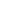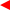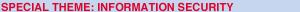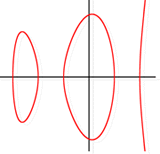contentsERCIM News No.49, April 2002A hyperelliptic curve over the real numbers. For cryptographic purposes one considers only curves over finite fields.# Cryptography using Hyperelliptic Curves

by Norbert Göb and Georg Kux

The Fraunhofer Institute for Industrial Mathematics in Kaiserslautern is developing a cryptosystem based on hyperelliptic curves. The main goals of this project are the establishment of the algorithmic foundations and the implementation of a prototype.

Nowadays the most popular public key cryptosystem is RSA. Its security relies on the fact that factoring a large integer known to be the product of two primes of similar size is supposed to be a computationally difficult problem. Nevertheless, good progress has been made in factoring due to the so-called number field sieve algorithm. The actual factoring record lies at 158 decimal digits for the composite number (see article in this issue), which means that 512-bit RSA-keys can no longer guarantee security.

Considering this development it is important to have alternatives available. Many proposals have been made, most of which are not comparable with RSA in terms of running time and security per key-length. Elliptic curves, however, have proved to be a good choice for building public key cryptosystems which can seriously compete with RSA. Their security relies on the problem of computing logarithms in the group of points of an elliptic curve. Since this is supposed to be hard for relatively small parameters, they offer high-grade security even for small keys and are therefore the optimal choice for smart cards and other environments that provide only a limited storage space. Hyperelliptic curves are generalisations of elliptic curves, ie, they are of a higher genus (elliptic curves have a genus equal to one). Not as much is known about them, but they can be used to construct secure and efficient cryptosystems comparable to RSA.

In our project we investigate how the parameters must be chosen to achieve this goal. Certainly this implies consideration of many theoretical results. Contrary to an elliptic curve, the set of points on a hyperelliptic curve alone does not allow a mathematical group structure. In this case one has to generalise somewhat to find a group that provides similar features. This is called the Jacobi group. To offer reasonable security it is of great importance to know the exact number of elements of this group, the so-called class number. This cannot be simply read from the curve equation and there is still no known efficient algorithm to compute it for a general hyperelliptic curve. For special classes of curves, however, there exist methods for determining the class number.

Our current research concentrates on finding construction methods for secure Jacobi groups. These include specifying algorithms to test whether the curve resists all known attacks. Another task is to speed up the arithmetic needed in order to improve the running times of the corresponding cryptosystem.